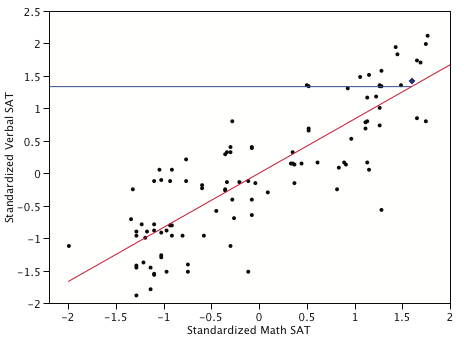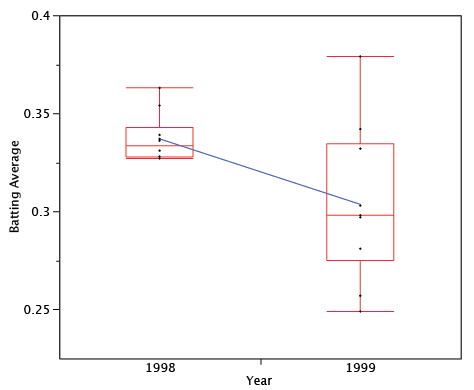# 10.7: Regression Toward the Mean

•• David Lane
• Rice University
$$\newcommand{\vecs}{\overset { \rightharpoonup} {\mathbf{#1}} }$$ $$\newcommand{\vecd}{\overset{-\!-\!\rightharpoonup}{\vphantom{a}\smash {#1}}}$$$$\newcommand{\id}{\mathrm{id}}$$ $$\newcommand{\Span}{\mathrm{span}}$$ $$\newcommand{\kernel}{\mathrm{null}\,}$$ $$\newcommand{\range}{\mathrm{range}\,}$$ $$\newcommand{\RealPart}{\mathrm{Re}}$$ $$\newcommand{\ImaginaryPart}{\mathrm{Im}}$$ $$\newcommand{\Argument}{\mathrm{Arg}}$$ $$\newcommand{\norm}{\| #1 \|}$$ $$\newcommand{\inner}{\langle #1, #2 \rangle}$$ $$\newcommand{\Span}{\mathrm{span}}$$ $$\newcommand{\id}{\mathrm{id}}$$ $$\newcommand{\Span}{\mathrm{span}}$$ $$\newcommand{\kernel}{\mathrm{null}\,}$$ $$\newcommand{\range}{\mathrm{range}\,}$$ $$\newcommand{\RealPart}{\mathrm{Re}}$$ $$\newcommand{\ImaginaryPart}{\mathrm{Im}}$$ $$\newcommand{\Argument}{\mathrm{Arg}}$$ $$\newcommand{\norm}{\| #1 \|}$$ $$\newcommand{\inner}{\langle #1, #2 \rangle}$$ $$\newcommand{\Span}{\mathrm{span}}$$$$\newcommand{\AA}{\unicode[.8,0]{x212B}}$$

Learning Objectives

• To study Regression toward the mean

Regression toward the mean involves outcomes that are at least partly due to chance. We begin with an example of a task that is entirely chance: Imagine an experiment in which a group of $$25$$ people each predicted the outcomes of flips of a fair coin. For each subject in the experiment, a coin is flipped $$12$$ times and the subject predicts the outcome of each flip. Figure $$\PageIndex{1}$$ shows the results of a simulation of this "experiment." Although most subjects were correct from $$5$$ to $$8$$ times out of $$12$$, one simulated subject was correct $$10$$ times. Clearly, this subject was very lucky and probably would not do as well if he or she performed the task a second time. In fact, the best prediction of the number of times this subject would be correct on the retest is $$6$$, since the probability of being correct on a given trial is $$0.5$$ and there are $$12$$ trials.Figure $$\PageIndex{1}$$: Histogram of results of a simulated experiment

More technically, the best prediction for the subject's result on the retest is the mean of the binomial distribution with $$N = 12$$ and $$p = 0.50$$. This distribution is shown in Figure $$\PageIndex{2}$$ and has a mean of $$6$$.Figure $$\PageIndex{2}$$: Binomial Distribution for $$N = 12$$ and $$p = 0.50$$

The point here is that no matter how many coin flips a subject predicted correctly, the best prediction of their score on a retest is $$6$$.

Now we consider a test we will call "Test A" that is partly chance and partly skill: Instead of predicting the outcomes of $$12$$ coin flips, each subject predicts the outcomes of $$6$$ coin flips and answers $$6$$ true/false questions about world history. Assume that the mean score on the $$6$$ history questions is $$4$$. A subject's score on Test A has a large chance component but also depends on history knowledge. If a subject scored very high on this test (such as a score of $$10/12$$), it is likely that they did well on both the history questions and the coin flips. If this subject is then given a second test (Test B) that also included coin predictions and history questions, their knowledge of history would be helpful and they would again be expected to score above the mean. However, since their high performance on the coin portion of Test A would not be predictive of their coin performance on Test B, they would not be expected to fare as well on Test B as on Test A. Therefore, the best prediction of their score on Test B would be somewhere between their score on Test A and the mean of Test B. This tendency of subjects with high values on a measure that includes chance and skill to score closer to the mean on a retest is called "$$\textit{regression toward the mean}$$."

The essence of the regression-toward-the-mean phenomenon is that people with high scores tend to be above average in skill and in luck and that only the skill portion is relevant to future performance. Similarly, people with low scores tend to be below average in skill and luck, and their bad luck is not relevant to future performance. This does not mean that all people who score high have above average luck. However, on average they do.

Almost every measure of behavior has a chance and a skill component to it. Take a student's grade on a final exam as an example. Certainly, the student's knowledge of the subject will be a major determinant of his or her grade. However, there are aspects of performance that are due to chance. The exam cannot cover everything in the course and therefore must represent a subset of the material. Maybe the student was lucky in that the one aspect of the course the student did not understand well was not well-represented on the test. Or, maybe the student was not sure which of two approaches to a problem would be better but, more or less by chance, chose the right one. Other chance elements come into play as well. Perhaps the student was awakened early in the morning by a random phone call, resulting in fatigue and lower performance. And, of course, guessing on multiple choice questions is another source of randomness in test scores.

There will be regression toward the mean in a test-retest situation whenever there is less than a perfect ($$r = 1$$) relationship between the test and the retest. This follows from the formula for a regression line with standardized variables shown below:

$Z_{Y'} = (r)(Z_X)$

From this equation it is clear that if the absolute value of $$r$$ is less than $$1$$, then the predicted value of $$Z_Y$$ will be closer to $$0$$, the mean for standardized scores, than is $$Z_X$$. Also, note that if the correlation between $$X$$ and $$Y$$ is $$0$$, as it would be for a task that is all luck, the predicted standard score for $$Y$$ is its mean, $$0$$, regardless of the score on $$X$$.

Figure $$\PageIndex{3}$$ shows a scatter plot with the regression line predicting the standardized Verbal SAT from the standardized Math SAT. Note that the slope of the line is equal to the correlation of $$0.835$$ between these variables.Figure $$\PageIndex{3}$$: Prediction of Standardized Verbal SAT from Standardized Math SAT

The point represented by a blue diamond has a value of $$1.6$$ on the standardized Math SAT. This means that this student scored $$1.6$$ standard deviations above the mean on Math SAT. The predicted score is $$(r)(1.6) = (0.835)(1.6) = 1.34$$. The horizontal line on the graph shows the value of the predicted score. The key point is that although this student scored $$1.6$$ standard deviations above the mean on Math SAT, he or she is only predicted to score $$1.34$$ standard deviations above the mean on Verbal SAT. Thus, the prediction is that the Verbal SAT score will be closer to the mean of $$0$$ than is the Math SAT score. Similarly, a student scoring far below the mean on Math SAT will be predicted to score higher on Verbal SAT.

Regression toward the mean occurs in any situation in which observations are selected on the basis of performance on a task that has a random component. If you choose people on the basis of their performance on such a task, you will be choosing people partly on the basis of their skill and partly on the basis of their luck on the task. Since their luck cannot be expected to be maintained from trial to trial, the best prediction of a person's performance on a second trial will be somewhere between their performance on the first trial and the mean performance on the first trial. The degree to which the score is expected to "regress toward the mean" in this manner depends on the relative contributions of chance and skill to the task: the greater the role of chance, the more the regression toward the mean.

## Errors Resulting From Failure to Understand Regression Toward the Mean

Regression toward the mean is frequently present in sports performance. A good example is provided by Schall and Smith ($$2000$$), who analyzed many aspects of baseball statistics including the batting averages of players in $$1998$$. They chose the $$10$$ players with the highest batting averages (BAs) in $$1998$$ and checked to see how well they did in $$1999$$. According to what would be expected based on regression toward the mean, these players should, on average, have lower batting averages in $$1999$$ than they did in $$1998$$. As can be seen in Table $$\PageIndex{1}$$, $$7/10$$ of the players had lower batting averages in $$1999$$ than they did in $$1998$$. Moreover, those who had higher averages in $$1999$$ were only slightly higher, whereas those who were lower were much lower. The average decrease from $$1998$$ to $$1999$$ was $$33$$ points. Even so, most of these players had excellent batting averages in $$1999$$ indicating that skill was an important component of their $$1998$$ averages.

Table $$\PageIndex{1}$$: How the Ten Players with the Highest BAs in $$1998$$ did in $$1999$$
1998 1999 Difference
363 379 16
354 298 -56
339 342 3
337 281 -56
336 249 -87
331 298 -33
328 297 -31
328 303 -25
327 257 -70
327 332 5

Figure $$\PageIndex{4}$$ shows the batting averages of the two years. The decline from $$1998$$ to $$1999$$ is clear. Note that although the mean decreased from $$1998$$, some players increased their batting averages. This illustrates that regression toward the mean does not occur for every individual. Although the predicted scores for every individual will be lower, some of the predictions will be wrong.Figure $$\PageIndex{4}$$: Quantile plots of the batting averages. The line connects the means of the plots.

Regression toward the mean plays a role in the so-called "Sophomore Slump," a good example of which is that a player who wins "rookie of the year" typically does less well in his second season. A related phenomenon is called the Sports Illustrated Cover Jinx.

An experiment without a control group can confound regression effects with real effects. For example, consider a hypothetical experiment to evaluate a reading-improvement program. All first graders in a school district were given a reading achievement test and the $$50$$ lowest-scoring readers were enrolled in the program. The students were retested following the program and the mean improvement was large. Does this necessarily mean the program was effective? No, it could be that the initial poor performance of the students was due, in part, to bad luck. Their luck would be expected to improve in the retest, which would increase their scores with or without the treatment program.

For a real example, consider an experiment that sought to determine whether the drug propranolol would increase the SAT scores of students thought to have test anxiety. Propranolol was given to $$25$$ high school students chosen because IQ tests and other academic performance indicated that they had not done as well as expected on the SAT. On a retest taken after receiving propranolol, students improved their SAT scores an average of $$120$$ points. This was a significantly greater increase than the $$38$$ points expected simply on the basis of having taken the test before. The problem with the study is that the method of selecting students likely resulted in a disproportionate number of students who had bad luck when they first took the SAT. Consequently, these students would likely have increased their scores on a retest with or without the propranolol. This is not to say that propranolol had no effect. However, since possible propranolol effects and regression effects were confounded, no firm conclusions should be drawn.

Randomly assigning students to either the propranolol group or a control group would have improved the experimental design. Since the regression effects would then not have been systematically different for the two groups, a significant difference would have provided good evidence for a propranolol effect.

New York Times Article on the Study

Schall, T., & Smith, G. (2000) Do Baseball Players Regress Toward the Mean? The American Statistician, 54, 231-235.

This page titled 10.7: Regression Toward the Mean is shared under a Public Domain license and was authored, remixed, and/or curated by David Lane via source content that was edited to the style and standards of the LibreTexts platform; a detailed edit history is available upon request.